# AY2021 June Revision P&C Solutions1. The two empty seats have to be considered as two identical objects, henceways.

2.3.or4.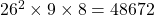5. (a)5. (b);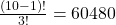6. (i)or6. (ii)6. (iii) Case 1: 4 distinct letters.Case 2: Exactly 3 distinct letters.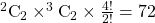Case 3: Exactly 2 distinct letters.Total number of ways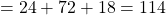7. (a) He has two options per coin, thus7. (b) He has four options, two options and 3 options for each denomination respectively, thus8. (i) If two particular canisters must not be placed on any of the 4 bases, then8. (ii) If two particular canisters must not be placed next to each other on the same side, then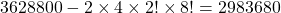9. (i)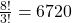9. (ii)9. (iii) Observe there are only two possible cases: D_G_T_S_ or _D_G_T_S.

Total number of ways9. (iv) Observe there are four possible cases: I _ _ I _ _ _ I // I _ _ _ I _ _ I // _ I _ _ I _ _ I // I _ _ I _ _ I _

Total number of ways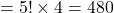10. (i) Case 1: First digit is ‘1’ or ‘2’.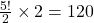Case 2: First digit is ‘3’:Total number of ways10. (ii) Case 1: First digit is ‘1’ and last digit is ‘1’:Case 2: First digit is ‘1’ and last digit is ‘3’:Case 3: First digit is ‘2’ and last digit is ‘3’:Case 4: First digit is ‘2’ and last digit is ‘1’:Total number of ways11. (a)11. (bi) Method 1: Case 1: all distinct:Case 2: all same:Case 3: AAB in any order:Total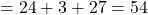Method 2: Case 1: Nobody get hazelnut: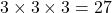Case 2: Somebody gets hazelnut:Total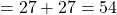11. (bii) Method 1: Complement

Number of ways a particular friend gets the hazelnut barRequired number of waysMethod 2: Direct

Case 1: Nobody gets hazelnut:Case 2: Somebody gets hazelnut:Total12. (i) Case 1: First digit is ‘3’, last digit can be ‘1’, ‘3’, or ‘5’:Case 2: First digit is ‘4’, last digit can be ‘1’, ‘3’, or ‘5’:Case 3: First digit is ‘5’, last digit can be ‘1’ or ‘3’:Number of ways12. (ii)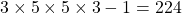13. (i)13. (ii)13. (iii)(7-1)!7! = 3628800\frac{^6 C_3 \times [(3-1)!]^2 \times 6!}{2} = 28800 \$

Not readable? Change text.